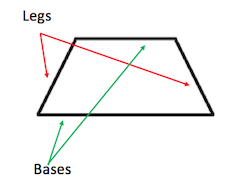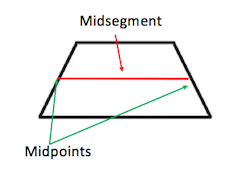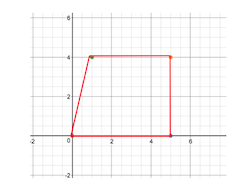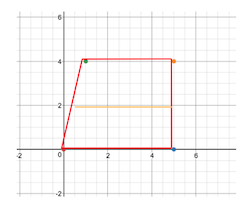# Using the Trapezoid Midsegment Theorem

Lesson Transcript
Instructor: Stephanie Matalone

Stephanie taught high school science and math and has a Master's Degree in Secondary Education.

In this lesson, we will discuss the basic definition of a trapezoid and its midsegment. Then, we will review the trapezoid midsegment theorem and go over some examples of how it is used. Updated: 04/14/2021

## Trapezoids

Are you a quadrilateral? Do you have a pair of parallel sides? Do you have another pair of sides that aren't parallel? If you answered ''yes'' to all of these questions, then our judges have determined that you are a trapezoid!

Trapezoids are 4-sided figures (quadrilaterals) with a pair of sides that are parallel and another pair that aren't. Parallel simply means that the sides are equal distance apart the whole way across, like train tracks. Starting at the middle, you can travel along them either way and see that the distance between the lines stays the same.

In a trapezoid, you can see that lines on the top and bottom of the trapezoid are parallel, while the lines on the sides are not parallel.

An error occurred trying to load this video.

Try refreshing the page, or contact customer support.

Coming up next: Kites in Geometry: Definition and Properties

### You're on a roll. Keep up the good work!

Replay
Your next lesson will play in 10 seconds
• 0:04 Trapezoids
• 0:46 The Midsegment
• 1:09 On a Coordinate Plane
• 1:59 Trapezoid Midsegment Theorem
• 2:38 Some Examples
• 4:12 Lesson Summary
Save Save

Want to watch this again later?

Timeline
Autoplay
Autoplay
Speed Speed## The Midsegment

Let's refer to the two sides of the triangle that aren't parallel as the legs. The other two sides that are parallel will be called the bases.The midsegment of a trapezoid is a line that goes across from the middle of one leg to the middle of the other leg. The midsegment meets each leg at the midpoint. The midpoint is equal distance from both ends of the lines of the legs.## On a Coordinate Plane

It is possible to use all of what we learned when we have a trapezoid on a coordinate plane. Let's say you are given the following four points:

• (0, 0) (5, 0) (1, 4) (5, 4)

You can graph those on a coordinate plane, drawing lines between the points to find that it makes a trapezoid.This trapezoid looks a little different than the one we saw earlier, but you can still see that the bases are parallel and the legs are not. We can easily draw in our midsegment here. Remember that the midsegment must start at the midpoints of each leg.

Since both of our legs start at 0 on the y-axis and end at 4 on the y-axis, it's easy to see that the midpoints should fall at 2 on the y-axis for both legs. Then we just draw our line across the see the midsegment.## Trapezoid Midsegment Theorem

Now that we understand some of the basics of trapezoids, let's talk about the trapezoid midsegment theorem, which states that the length of the midsegment is equal to the sum of the base lengths divided by 2. In other words, the midsegment is the average length of the two bases.

So let's say we are looking at a trapezoid where the length of the base on top is 2 and the length of the base on the bottom is 4. To find the length of the midsegment, we would simply add up the lengths of the bases:

• 2 + 4 = 6

Then divide the sum by 2:

• 6 / 2 = 3

To unlock this lesson you must be a Study.com Member.

### Register to view this lesson

Are you a student or a teacher?